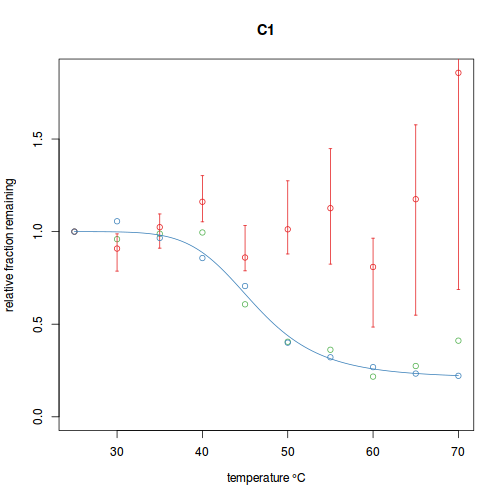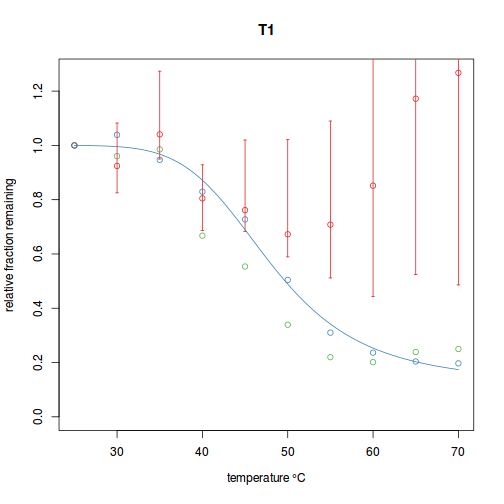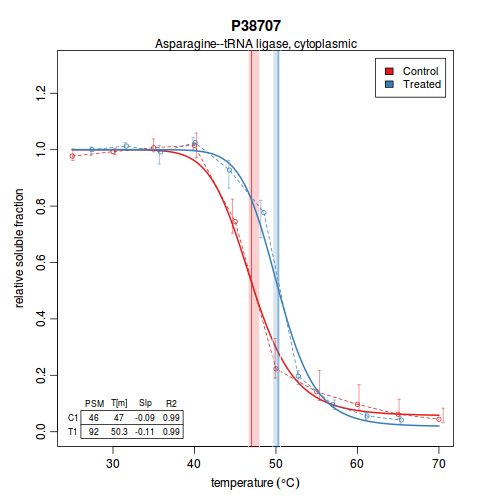# MSTherm

The mstherm package is used for modeling and analysing thermal proteome profiling (TPP) experiments. For more details on the motivation and methodology behind such experiments, please see Savitski et al, 2014. Analyzing a TPP experiment in mstherm involves generating table of quantative temperature series data for each replicate, setting up metadata files describing the experiment, creating an MSThermExperiment object, normalizing the data, modeling, visualization, and data export. Each of these steps will be described below as a sample dataset is analyzed.

## Input files

### Spectral data

The primary input to mstherm is a set of tab-delimited data tables containing quantative data from the experiment, one file per replicate. The table consists of a header line followed by one line per peptide spectral match. It can contain any number of columns, but certain columns must be present (and labeled properly) to be used with mstherm. Specifically, the folowing columns are required to be present:

• peptide - the sequence of the matched peptide in single-letter IUPAC
• protein - the protein group the peptide is assigned to
• ... - one column per temperature point, with labels matching those in the meta table described below

The following columns are optional but contain information that can be used for filtering (all other columns are simply ignored):

• score - the score assigned by the search engine or processing software (e.g. Percolator, PeptideProphet) to the peptide spectral match
• coelute_inf - the fraction of the signal in the precursor window derived from off-target ions (e.g. the percent co-isolation interference, from 0.0-1.0)

These data files are generally produced by whatever quantification software is used, but may need manual or batch processing to apply the correct column labels.

Although mstherm has some capabilities for reading metadata from existing dataframes, typically this information will be read from files on disk. While these files can be arranged in any way the user wishes, it is recommended that a new directory be set up for each project with the following layout:

├── control.tsv
├── data
│   ├── Control_R1.tsv
│   ├── Control_R2.tsv
│   ├── Control_R3.tsv
│   ├── Treated_R1.tsv
│   ├── Treated_R2.tsv
│   └── Treated_R3.tsv
└── meta
└── tags.meta


The control.tsv file is a tab-delimited table describing the experiment and paths to relevant files. An example of a control file for the above experiment would be as follows:

name    sample  data_file   meta_file
C1  Control data/Control_R1.tsv meta/tags.meta
C2  Control data/Control_R2.tsv meta/tags.meta
C3  Control data/Control_R3.tsv meta/tags.meta
T1  Treated data/Treated_R1.tsv meta/tags.meta
T2  Treated data/Treated_R2.tsv meta/tags.meta
T3  Treated data/Treated_R3.tsv meta/tags.meta


Paths in this file are relative to the location of the control file itself. In this experiment, all samples used the same quantitative methodology and so all share the same meta file, but any number of meta files can be used. The meta_file is another tab-delimited table that maps quantitative column labels in the data files to temperature points. An example for an experiment utilizing TMT-10plex isobaric labeling might look as follows:

channel temp
TMT10.126   28.2
TMT10.127N  31.3
TMT10.127C  35.0
TMT10.128N  38.5
TMT10.128C  42.1
TMT10.129N  45.7
TMT10.129C  49.1
TMT10.130N  52.2
TMT10.130C  56.2
TMT10.131   59.4


The channel labels can be anything the user chooses as long as they match with the appropriate columns in the data file. In all of these metadata files, the column labels must match those shown above.

### Annotations

If available, a tab-delimited table containing descriptions for each protein group can be imported and used for plot labels during visualization. This table should have two columns labeled name and annotation.

## Initializing an analysis

With the data files in hand as described, an mstherm session is started as follows:

library(mstherm)
control <- system.file("extdata", "demo_project/control.tsv", package="mstherm")
annots <- system.file("extdata", "demo_project/annots.tsv", package="mstherm")
expt <- MSThermExperiment(control, annots)


This will read the control file and load all necessary quantitative data and metadata into the MSThermExperiment object. This is the step in which the table of annotations can be provided if available (this information is only used to provide descriptive labels on protein melting plots). Here we are using files included in the demo of this package.

## Normalization

Typically, after importing the raw quantitiative data a normalization step is performed to correct for differences in sampling handling and other sources of variance that can introduce variance from channel to channel. There are two methods available in mstherm to perform this step, normalize_to_std() and normalize_to_profile(). normalize_to_std() requires that a protein spike-in be added equally to all samples directly after gradient precipitation, and the method calculates and applies scaling factors to each channel such that the values for the spike-in are roughly equal across channels. The normalization is applied independently to each replicate. In the demo data, BSA was spiked in to each sample, and we use it here to apply the normalization method (using the protein ID which matches that used in the data tables):

expt <- normalize_to_std(expt, "cRAP_ALBU_BOVIN")This performs the normalization as well as producing a summary plot for QC purposes (which can be captured to PDF, etc, by changing the output device before the above step). In the plots above, green points represent the original channel intensity sums (relative to the first temperature), the red points show relative quantification of spike-in standard pre-normalization (with IQR indicated by red bars), the blue points show normalized relative channel sums, and the blue curve is final normalization curve used to generate scaling factors.

The other available normalization method, normalize_to_profile(), takes a vector of values equal in length to the number of quantification channels and calculates and applies scaling factors to each channel such that the ratios between sums of quantification values for each channel match the ratios between values given in the vector. Such values might correspond to, for example, measured total protein concentration in each sample after gradient precipitation.

## Modeling

The temperature series data is attempted to be fit to a logistic curve model for two-state unfolding according the following formula:

$Pn = \frac{1 - \textit{p}}{1 + e^{-k(\frac{1}{T}-\frac{1}{m})}} + \textit{p}$

where $$T$$ is the temperature and $$m$$, $$k$$, and $$p$$ are estimated parameters ($$m$$ corresponds to the melting temperature, $$p$$ the lower plateau of the curve, and $$k$$ contributes to the slope of the curve).

Modeling of the data is performed with the following command:

res <- model_experiment(expt, bootstrap=T, smooth=T, min_rep_psm=3, np=1)


There are many, many options available to this command which control various aspects of modeling and filtering (see the documentation for full details). As modeling can be slow (especially if you have data for several thousand proteins and bootstrapping is turned on), the package can utilize parallel processing to speed things up. This is set by the np parameter, which by default will use all available threads on the machine.

## Inter-replicate normalization

A second round of normalization can be performed to correct for differences in sample handling between replicates in experiments where most proteins are not expected to be changing and the global distribution of protein Tms is expected to be constant. Doing so requires that the dataset first be modeled as above to estimate Tms for each protein. A single replicate is then chosen as the baseline and correction factors are calculated for all other replicates based on linear regression between a set of high-quality melting temperature estimates. These linear corrections are applied to the intial temperature vector for each replicate and a new MSThermExperiment object is returned which can be re-modeled as above. The command to perform this inter-replicate normalization is:

expt <- normalize_to_tm( expt, res )
res  <- model_experiment(expt, bootstrap=T, smooth=T, min_rep_psm=3, np=1)


## Visualization

Melting plots can be produced for each protein in the result set. This can be done either for an individual protein or for the whole set at once. For example, the following command will generate a plot for protein P38707 of the test data:

plot(res\$P38707)Again, there are a number of options that can be passed to the plot() command to control what is shown on the plots (by default, most options are turned on). The plot() command can also be passed a list of proteins to plot, or no arguments at all (in which case all proteins are plotted sequentially). For example, the following will produce a PDF file with each protein plot on a separate page:

pdf("models.pdf", 5, 5, pointsize=10, useDingbats=F)
plot(res)
dev.off()


## Data Export

The as.data.frame() method produces a summary dataframe containing melting temperatures, model parameter estimates, and other descriptive values for each protein that can be used for further analysis in R or written to file using the usual methods (e.g. write.table()). The output from this command contains the following columns (row labels are protein group IDs):

• annotation - taken from annotation table, if provided, or else empty
• for each replicate in the experiment:
• tm - melting temperature (the $$m$$ parameter in the model)
• psm - number of PSMs used for protein-level quantification
• inf - protein-level estimate of co-isolation interference
• slope - slope of the model at the inflection point
• k - the $$k$$ parameter estimate of the model
• plat - the $$p$$ or “plateau” parameter estimate of the model
• r2 - the coefficient of determination of the model fit
• rmsd - the root-mean-square deviation of the model fit